JEE  >  Test: Single Correct MCQs: Motion | JEE Advanced

# Test: Single Correct MCQs: Motion | JEE Advanced

Test Description

## 12 Questions MCQ Test Physics For JEE | Test: Single Correct MCQs: Motion | JEE Advanced

Test: Single Correct MCQs: Motion | JEE Advanced for JEE 2022 is part of Physics For JEE preparation. The Test: Single Correct MCQs: Motion | JEE Advanced questions and answers have been prepared according to the JEE exam syllabus.The Test: Single Correct MCQs: Motion | JEE Advanced MCQs are made for JEE 2022 Exam. Find important definitions, questions, notes, meanings, examples, exercises, MCQs and online tests for Test: Single Correct MCQs: Motion | JEE Advanced below.
Solutions of Test: Single Correct MCQs: Motion | JEE Advanced questions in English are available as part of our Physics For JEE for JEE & Test: Single Correct MCQs: Motion | JEE Advanced solutions in Hindi for Physics For JEE course. Download more important topics, notes, lectures and mock test series for JEE Exam by signing up for free. Attempt Test: Single Correct MCQs: Motion | JEE Advanced | 12 questions in 25 minutes | Mock test for JEE preparation | Free important questions MCQ to study Physics For JEE for JEE Exam | Download free PDF with solutions
 1 Crore+ students have signed up on EduRev. Have you?
Test: Single Correct MCQs: Motion | JEE Advanced - Question 1

### A river is flowing from west to  east at a speed of 5 metres per minute. A man on the south bank of the river, capable of swimming at 10 metres per minute in still water, wants to swim across the river in the shortest time. He should swim in a direction

Detailed Solution for Test: Single Correct MCQs: Motion | JEE Advanced - Question 1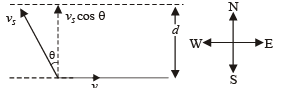Time taken to cross the river t = d/v5cosθ

NOTE :  For time to be minimum, cosθ = maximum⇒θ= 0°

The swimmer should swim due north.

Test: Single Correct MCQs: Motion | JEE Advanced - Question 2

### A boat which has a speed of 5 km/hr in still water crosses a river of width 1 km along the shortest possible path in 15 minutes. The velocity of the river water in km/hr is

Detailed Solution for Test: Single Correct MCQs: Motion | JEE Advanced - Question 2

Shortest route corresponds to vrb perpendicular to river flow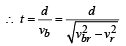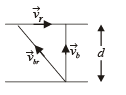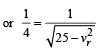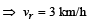Test: Single Correct MCQs: Motion | JEE Advanced - Question 3

### In 1.0 s, a particle goes from point A to point B, moving in a semicircle of radius 1.0 m (see Figure). The magnitude of the average velocity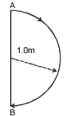Detailed Solution for Test: Single Correct MCQs: Motion | JEE Advanced - Question 3

| Average velocity | =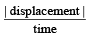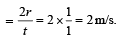Test: Single Correct MCQs: Motion | JEE Advanced - Question 4

A ball is dropped vertically from a height d above the ground.
It hits the ground and bounces up vertically to a height d/2.
Neglecting subsequent motion and air resistance, its velocity v varies with the height h above the ground as

Detailed Solution for Test: Single Correct MCQs: Motion | JEE Advanced - Question 4

(a) KEY CONCEPT Before hitting the ground, the velocity v is given by v2 = 2 gd (quadratic equation and hence parabolic path) Downwards direction means negative velocity. After collision, the direction become positive and velocity decreases.
Further,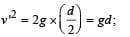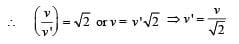Test: Single Correct MCQs: Motion | JEE Advanced - Question 5

A particle starts sliding down a frictionless inclined plane.
If Sn is the distance travelled by it from time t = n – 1 sec to t = n sec, the rati o Sn/Sn+1 is

Detailed Solution for Test: Single Correct MCQs: Motion | JEE Advanced - Question 5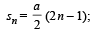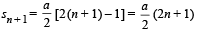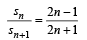Test: Single Correct MCQs: Motion | JEE Advanced - Question 6

A body starts from rest at time t = 0, the acceleration time graph is shown in the figure. The maximum velocity attained by the body will be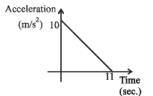Detailed Solution for Test: Single Correct MCQs: Motion | JEE Advanced - Question 6

Change in velocity = area under the graph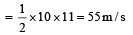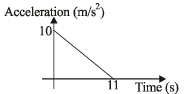Since, initial velocity is zero, final velocity is 55 m/s.

Test: Single Correct MCQs: Motion | JEE Advanced - Question 7

The velocity-displacement graph of a particle moving along a straight line is shown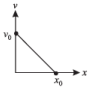The most suitable acceleration-displacement graph will be

Detailed Solution for Test: Single Correct MCQs: Motion | JEE Advanced - Question 7

The equation for the given v-x graph is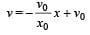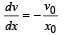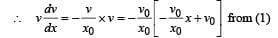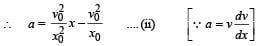On comparing the equation (ii) with equation of a straight line

y = mx + c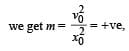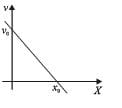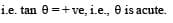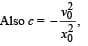i.e., they-intercept is negative The above conditions are satisfied in graph (a).

Test: Single Correct MCQs: Motion | JEE Advanced - Question 8

Two identical discs of same radius R are rotating about their axes in opposite directions with the same constant angular speed w. The discs are in the same horizontal plane. At time t = 0, the points P and Q are facing each other as shown in the figure. The relative speed between the two points P and Q is vr. In one time period (T) of rotation of the discs, vr as a function of time is best represented by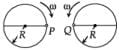Detailed Solution for Test: Single Correct MCQs: Motion | JEE Advanced - Question 8

At t = 0,  the relative velocity will be zero.

At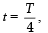,  the relative velocity will be maximum in magnitude.

At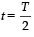,  the relative velocity will be zero.

At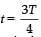,  the relative velocity will be maximum in magnitude

At t = T, the relative velocity again becomes zero.

Test: Single Correct MCQs: Motion | JEE Advanced - Question 9

Consider a disc rotating in the horizontal plane with a constant angular speed w about its centre O. The disc has a shaded region on one side of the diameter and an unshaded region on the other side as shown in the figure. When the disc is in the orientation as shown, two pebbles P and Q are simultaneously projected at an angle towards R. The velocity of projection is in the y-z plane and is same for both pebbles with respect to the disc. Assume that (i) they land back on the disc before the disc has completed 1/8 rotation, (ii) their range is less than half the disc radius, and (iii) w remains constant throughout. Then .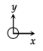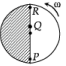Detailed Solution for Test: Single Correct MCQs: Motion | JEE Advanced - Question 9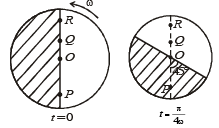The x-coordinate of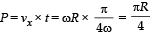This horizontal distance travelled will be greater than any point on the disc between O and P. Therefore the landing will be in unshaded area. In the same way, the horizontal distance travelled by Q is always less than that of any point between O and R. Therefore the landing will be in unshaded area.

*Multiple options can be correct
Test: Single Correct MCQs: Motion | JEE Advanced - Question 10

A particle is moving eastwards with a velocity of 5 m/s. In 10s the velocity changes to 5 m/s northwards. The average acceleration in this time is

Detailed Solution for Test: Single Correct MCQs: Motion | JEE Advanced - Question 10

Average acceleration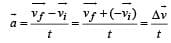To find the resultant of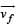and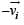, we draw a diagram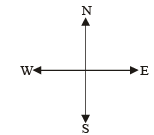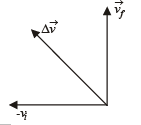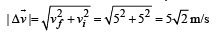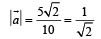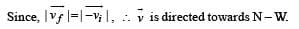*Multiple options can be correct
Test: Single Correct MCQs: Motion | JEE Advanced - Question 11

A particle of mass m moves on the x-axis as follows : it starts from rest at t = 0 from the point x = 0, and comes to rest at t = 1 at the point x = 1. NO other information is available about its motion at intermediate times (0 < t< 1). If a denotes the instantaneous acceleration of the particle, then:

Detailed Solution for Test: Single Correct MCQs: Motion | JEE Advanced - Question 11

Note : a cannot remain positive for all t in the interval 0 < t < 1. This is because since the body starts from rest, it will first accelerate. Finally it stops therefore a will become negative. Therefore a will change its direction. Options (a) and (d) are correct.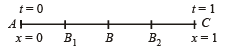Let the particle accelerate uniformly till half the distance (A to B) and then retard uniformly in the remaining half distance (B to C).
The total time is 1 sec. Therefore the time taken from A to B is 0.5 sec.
For A to B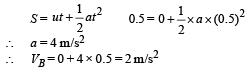Note :  Now, if the particle accelerates till B2 then for covering the same total distance in same time, acceleration should be less than 4 m/s2 but |deceleration| should be greater than 4 m/s2. And if the particle accelerates till B1, then for covering the same total distance in the same time, the acceleration should be greater than 4 m/s2 and | deceleration | < 4 m/s2.
The same is depicted by the graph.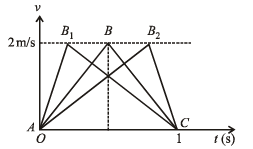So, the | acceleration | must be greater than or equal to 4 m/s2 at some point or points in the path.

*Multiple options can be correct
Test: Single Correct MCQs: Motion | JEE Advanced - Question 12

The coordinates of a particle moving in a plane are given by x(t) = a cos (pt) and y (t) = b sin (pt) where a, b (< a) and p are positive constants of appropriate dimensions. Then

Detailed Solution for Test: Single Correct MCQs: Motion | JEE Advanced - Question 12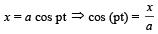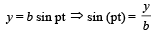Squaring and adding (1) and (2), we get,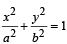∴ The path of the particle is an ellipse.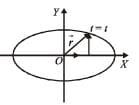From the given equations we can find,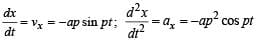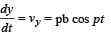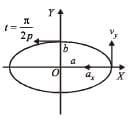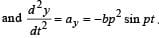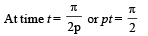ax and vy become zero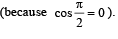Only
vx and ay are left, or we can say that velocity is along negative x-axis and acceleration along negative y-axis.

Hence, at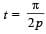velocity and acceleration of theparticle are normal to each other.

At t = t , position of the particle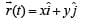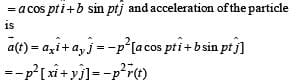Therefore, acceleration of the particle is always directed towards origin.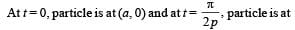(0, b). Therefore, the distance covered is one fourth of the elliptical path and not a.

## Physics For JEE

252 videos|559 docs|257 tests
 Use Code STAYHOME200 and get INR 200 additional OFF Use Coupon Code
Information about Test: Single Correct MCQs: Motion | JEE Advanced Page
In this test you can find the Exam questions for Test: Single Correct MCQs: Motion | JEE Advanced solved & explained in the simplest way possible. Besides giving Questions and answers for Test: Single Correct MCQs: Motion | JEE Advanced, EduRev gives you an ample number of Online tests for practice

## Physics For JEE

252 videos|559 docs|257 tests